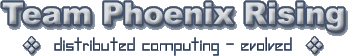# Excel help

Being trawling the web and tbh the web has confused me moreI am wanting to do permutations in Excel - initially I was going to go about it in one way but realised I would could end up with Billions of permutations.

I have realised since I can make a smaller data subset but then I need to kind of do the following I realise this is going to be a e.g. 65432*1 type sort

But I want to do exclusions & Recalc’s and not have a full list of pemutations

Example
…Col A----Col B-----Col C----Col D

1. Item1 - 30mins - Item2 - Recalculated figure
2. Item2 - 25mins - Item1 - Recalculated figure
3. Item3 - 45mins - Item3 - recalculated figure
4. Item4 - 30mins - Item4 - Recalculated figure
…Total - 130 --------------- recalculated figure

So Basically it would be all purmutation of column A & Column B is calculated value from another lookup.
In the background it would do its next permutation save to an array, recalculate the B column data from a lookup but not necesserily update excel table in col C & D.

If the recalculated total for the next permutation is less than that for sum of Column B total it would update Column C & D with the relivent data.

So I need to set the size of the Dataset so it knows how many line of data to use, do the permutations grab the recalculated figure if figure is less update table if not skip and do next permutation

Head fried :whiteflag: any help pointers much appreciated.
/Paul.

Basically this is a time optimisation type calculation to get to the lowest possible time.

No idea Paul sorry.Probably something that Kevin could help you with. He’s solved Excel probs for me in the past.

With all the temp cals Paul I think this would be better suited in a DB. Excel is great but when you have these many pointers to external sheets (not one) it tends to get harder to find an answer that will work quickly without making the system to slow. I would make it out maybe in ACCESS or SQL and look at the data layed out before jumping really deep into Excel.

I gave up on the ideaThe effort verse the gain was not worth it lol

I did a simple 10 depth loop permutation something like 3.8 Million calculations… it was still thinking about it 30 min later so terminated the macro.

That was not including any recalculation stuff just a brute force with simple numbers.

Certainly far too complex for Excel, I could handle it a different way but the effort to program it is just not worth it.